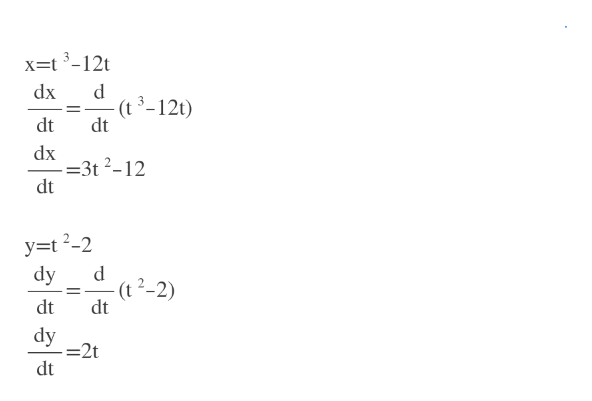# x = t3 − 12t,    y = t2 − 2findd2ydx2and for which values of t is the curve concave upward? (use interval notation).

Question

x = t3 − 12t,    y = t2 − 2

find

 d2y dx2

and for which values of t is the curve concave upward? (use interval notation).

check_circle

Step 1

We find dx/dt and dy/dt.help_outlineImage Transcriptionclosex=t 3-12t dx (t 3-12t) dt dt dx =3t 2-12 dt y=t?-2 dy (t 2-2) dt dt dy =2t dt fullscreen
Step 2

Using dx/dt and dy/dt we find dy/dx

Step 3

then we find the second derivative. Apply ...

### Want to see the full answer?

See Solution

#### Want to see this answer and more?

Solutions are written by subject experts who are available 24/7. Questions are typically answered within 1 hour.*

See Solution
*Response times may vary by subject and question.
Tagged in

### Other